EL10A TUTORIAL #1   Monday, 20 January 2003

1. Temperature measurements indicate that a heater has provided 1200 J of energy to a container of water in a time interval of 1 minute. The charge that has passed through the heater in this time interval is 100 C. What is the constant voltage across the heater ?

2. A direct lightning strike to the Cathedral of Learning at the University of Pittsburg was recorded on June 10, 1939

(a) The current from the cloud to ground is given by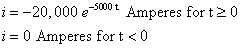What charge was transferred from the cloud to ground ?

(b) Just before the lightning struck, the electric field between the cloud base and ground was 10 kV/m, and the base of the cloud was 3 km above ground, determine the maximum voltage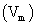of the cloud with respect to ground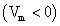just before the lightning struck.

(c) The instantaneous voltage of the cloud with respect to ground is given by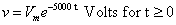determine the instantaneous power dissipated in the lightning strike.

(d) Determine the total energy dissipated in the lightning stike.

3. Calculate voltagesand currents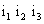for this network4. Replace the network to the right of terminals a and b in the network shown below with an equivalent circuit consisting of a single series resistance (R), series inductance (L) and series capacitance (C)5. Find i in the network below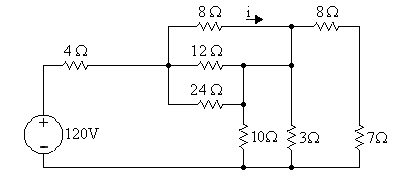6. Find v and i in the network below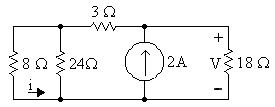7. Find v in the network below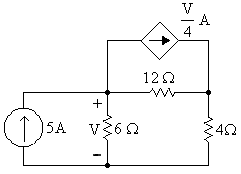EL10A Homepage